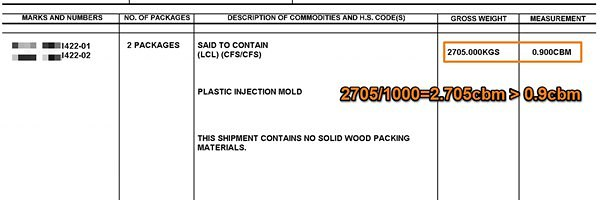# Understanding Volumetric Weight And How LCL Chargeable Weight Calculated

LCL stands for Less Than Container Load; it is a term used for shipments that make use of partial container freight. If an entire container is used for a single shipment (FCL or Full Container Load), the volume of the cargo becomes extraneous since the client owns the whole container, while carrier would have a weight limitation for FCL. However, in a case where the consignment is small, requiring only partial container freight, a universal formula is applied for calculating LCL chargeable weight.

What Is LCL Chargeable Weight?

Consider two shipments – one comprising of 1 ton of granite stones and the other 1 ton of cotton. Even though the two shipments weigh exactly the same the latter will occupy more space, and should logically be charged more.

This becomes more relevant with LCL shipments where there is no determining how much space a cargo might take. This is why LCL attracts different freight charges, which are determined based on the volume and weight of the packages.

Terms Used In Calculating LCL Ocean Freight

The terms used in the business can often be confusing for someone shipping goods for the first time. Freight forwarders often use terms such as dimensional weight or volumetric weight for calculating LCL ocean freight; these incidentally are different terms for determining the volume of the packages.

The dimensional or volumetric weight is measured in cubic meters (CBMs). For LCL cargo, freight charges are calculated on the basis of which of the two is greater; the actual weight of the cargo measured in metric tons; that is 1,000kgs or the volume in CBMs.

It must be noted here that when calculating ocean freight for LCL 1 Cubic Meter (CBM) is equal to 1 metric ton (1,000kgs). This means that any cargo with volumetric weight of 1 CBM would be considered as weighing 1 metric ton, and the freight charges would be levied accordingly. Conversely, if the volumetric weight is more than 1CBM then the freight for LCL is calculated based on the higher of the two values of the gross weight and CBM.

Freight forwarders also have a minimum chargeable weight of 1 metric ton or 1 CBM. The freight charges are levied for the minimum chargeable weight in case the shipment weighs less than 1 metric ton or 1 CBM.

Calculating CBM or Volumetric Weight

The formula for working out the volumetric weight/CBM is simple. You’ll need to take the length, width and height of all packages in meters and multiply them to obtain the CBM. So, the CBM of a package with length 1.5mtrs and width of 1.6mtrs and height of 2.7mtrs will be calculated
thus–
1.5 x 1.6 x 2.7 = 6.48CBM
If the measurements are obtained in centimeters then the formula for calculation will be –
Length x Width x Height/1,000,000 = CBM
However, if the measurements taken are in inches they will need to be converted into meters, to get the accurate CBM.

Examples of LCL Freight Charges –Gross Weight of Package – 2705kgs =2.705 metric tons
Measurement = 0.9CBM
Since the gross weight of 2.705 metric tons is greater than the CBM weight of 0.9cbm the chargeable weight will be calculated on the basis of the gross weight, that is 2.705 metric tons.

Gross Weight of Cargo = 2.705 metric tons
Measurement of cargo = 1.5 x 1.6 x 2.7 =6.48CBM
In this example the CBM is higher than the gross weight so the LCL chargeable weight will be the volumetric weight.

### Contact

#### +86 755 8368 1686

WhatsApp contact:

Skype contact:

### tsfreight

B1240, Tianxia International Center